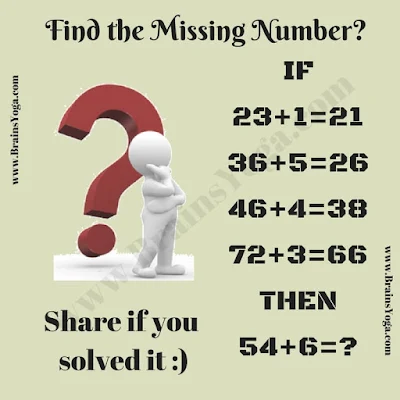How good is your logical reasoning? Let's check your logical reasoning with this Logic and Number Puzzle. Here are some logical equations. Find the logic in these equations and find the value of the missing number which will replace the question mark?Can you find the value of the number which replaces '?'?